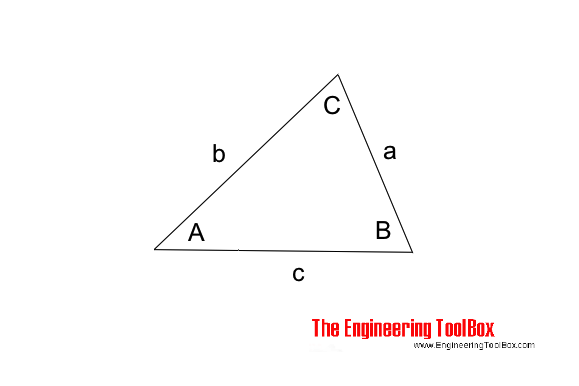Engineering ToolBox - Resources, Tools and Basic Information for Engineering and Design of Technical Applications!

# Law of Sines

## Calculate the angles in a generic triangle.

The "Law of Sines" can be used to calculate the angles of a triangle.The "Law of Sines" can be expressed as

$${a \over sin A } = {b \over sin B } = {c \over sin C } \tag{1}$$

where

a, b and c = length of sides in triangle (m, ft ...)

A, B and C = angles in the triangle (degrees)

### Law of Sines Calculator

a (m, ft ....)

b (m, ft ....)

A (degrees)

Eq. 1 can be modified to

$$a = {b sin A \over sin B } = {c sin A \over sin C } \tag{1a}$$

$$b = {a sin B \over sin A } = {c sin B \over sin C } \tag{1b}$$

$$c = {a sin C \over sin A } = {b sin C \over sin B } \tag{1c}$$

This expression can also be handy:

$$sin \frac{1}{2}A = \sqrt{{(s - b)(s - c) \over b c }} \tag{2}$$

where

$$s = \frac{1}{2}(a + b + c) \tag{3}$$

## Related Topics

• ### Mathematics

Mathematical rules and laws - numbers, areas, volumes, exponents, trigonometric functions and more.

## Related Documents

• ### Angle Calculator - Carpenter's Square

Calculate angles with a straight board across carpenter's square.
• ### Exponents - Powers and Roots

The laws of fractional and integer exponents.
• ### Factorials

The product of all positive integers.
• ### Fractions

Law of fractions
• ### Law of Cosines

One side of a triangle when the opposite angle and two sides are known.
• ### Law of Tangents

Triangles and law of tangents.
• ### Pythagorean Theorem

Verifying square corners.
• ### Right Angled Triangle

Right angled triangle equations.
• ### Squaring with Diagonal Measurements

A rectangle is square if the lengths of both diagonals are equal.
• ### Standard Differentials and Integrals

Equations for differentials and integrals.
• ### Taylor Series

Function as an infinite sum of terms.
• ### Triangle

Triangle analytical geometry.

## Engineering ToolBox - SketchUp Extension - Online 3D modeling!

Add standard and customized parametric components - like flange beams, lumbers, piping, stairs and more - to your Sketchup model with the Engineering ToolBox - SketchUp Extension - enabled for use with older versions of the amazing SketchUp Make and the newer "up to date" SketchUp Pro . Add the Engineering ToolBox extension to your SketchUp Make/Pro from the Extension Warehouse !

We don't collect information from our users. More about

## Citation

• The Engineering ToolBox (2013). Law of Sines. [online] Available at: https://www.engineeringtoolbox.com/law-of-sines-d_1858.html [Accessed Day Month Year].

Modify the access date according your visit.

9.19.12